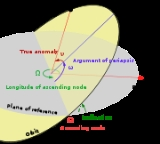xInclinationOverview

Inclination in general is the angle
Angle
In geometry, an angle is the figure formed by two rays sharing a common endpoint, called the vertex of the angle.Angles are usually presumed to be in a Euclidean plane with the circle taken for standard with regard to direction. In fact, an angle is frequently viewed as a measure of an circular arc...

between a reference plane
Plane of reference
A term used in celestial mechanics, the plane of reference is the plane by means of which orbital elements are defined. The two main orbital elements that are measured with respect to the plane of reference are the inclination and the longitude of the ascending node.Depending on the type of body...

and another plane or axis of direction.
The inclination is one of the six orbital parameters describing the shape and orientation of a celestial orbit
Orbit
In physics, an orbit is the gravitationally curved path of an object around a point in space, for example the orbit of a planet around the center of a star system, such as the Solar System...

. It is the angular
Angle
In geometry, an angle is the figure formed by two rays sharing a common endpoint, called the vertex of the angle.Angles are usually presumed to be in a Euclidean plane with the circle taken for standard with regard to direction. In fact, an angle is frequently viewed as a measure of an circular arc...

distance of the orbital plane from the plane of reference
Plane of reference
A term used in celestial mechanics, the plane of reference is the plane by means of which orbital elements are defined. The two main orbital elements that are measured with respect to the plane of reference are the inclination and the longitude of the ascending node.Depending on the type of body...

(usually the primary's equator
Equator
An equator is the intersection of a sphere's surface with the plane perpendicular to the sphere's axis of rotation and containing the sphere's center of mass....

or the ecliptic
Ecliptic
The ecliptic is the plane of the earth's orbit around the sun. In more accurate terms, it is the intersection of the celestial sphere with the ecliptic plane, which is the geometric plane containing the mean orbit of the Earth around the Sun...

), normally stated in degree
Degree (angle)
A degree , usually denoted by ° , is a measurement of plane angle, representing 1⁄360 of a full rotation; one degree is equivalent to π/180 radians...

s.

In the Solar System
Solar System
The Solar System consists of the Sun and the astronomical objects gravitationally bound in orbit around it, all of which formed from the collapse of a giant molecular cloud approximately 4.6 billion years ago. The vast majority of the system's mass is in the Sun...

, the inclination of the orbit of a planet
Planet
A planet is a celestial body orbiting a star or stellar remnant that is massive enough to be rounded by its own gravity, is not massive enough to cause thermonuclear fusion, and has cleared its neighbouring region of planetesimals.The term planet is ancient, with ties to history, science,...

is defined as the angle between the plane of the orbit of the planet and the ecliptic — which is the plane containing Earth
Earth
Earth is the third planet from the Sun, and the densest and fifth-largest of the eight planets in the Solar System. It is also the largest of the Solar System's four terrestrial planets...

's orbital path.